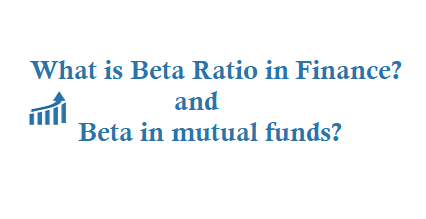# What is Beta Ratio in Finance? and What is Beta in mutual funds?

Beta ratio in finance is one of the most used ratios, and almost all of us have come across this term sometime. Few, however, understand what is beta ratio in mutual funds, or in finance in general, and what can we do with it. If you look at the Wikipedia page on Beta (Finance), it is scary enough for general investors.

With all kinds of graphs and formulae and Greek alphabets, it helps you little to understand the basics. Here, without going into much mathematics, we will see things as a normal investor; trying to understand what is beta ratio in stocks and mutual funds, why should we look at beta ratio while investing, and how not to misuse it. Keep reading!

# Beta Ratio in Finance

Definition of Beta in Finance: What does it mean?

Beta (β) in finance is a ratio that measures the volatility of a security against market volatility. If you want some technical language, Wikipedia gives you a nice definition. Beta in finance, it says, “is a number describing the correlated volatility of an asset in relation to the volatility of the benchmark that said asset is being compared to.”. In other words, the Beta ratio is used by Capital Asset Pricing Models (CAPM) to measure the risk of an asset, in relation to the market, or a benchmark. The default β ratio is 1. If an asset has 1 β ratio, its volatility (or risk and return) is at par with the market. If the β ratio is 2, the volatility is doubled.So, how do you use Beta ratio in personal finance?

What is the use of theoretical knowledge, if you cannot apply it? Let us see how to interpret several values of Beta ratio, and how to use it while making investment decisions.

Various possible values of Beta with interpretations

β < 0: If the value of beta is less than zero, that means the asset goes in opposite direction to the market movement. A good example can be gold, which goes in the opposite direction of stock market. That is why investing in gold can be a good option for hedging your overall portfolio.

β = 0: If beta is equal to zero that means the market index has no impact on the asset. A good example can be fixed income instruments like bank fixed deposits.

0 < β < 1: If beta ratio is between zero and one, that means the asset moves in the direction of the market index, but its risk and returns are lower than the benchmark. Long term debt mutual funds are good examples of this that have low risk and return scenario.

β = 1: If beta is one, the asset moves at par with the benchmark index. Large cap mutual funds are good examples of this.

β > 1: If Beta is more than 1, that means the asset is highly volatile and subject to steep fluctuations. Volatile stocks, small and mid cap mutual funds etc. are good examples.

## Beta in mutual funds

Why should you look at beta ratio while investing in mutual funds?

Be it stocks or mutual funds, understanding the risks vis-a-vis the return is essential. Market based investments like mutual funds are subject to risk, and you should take a look at the beta ratio before investing. If you have high or moderate risk taking capabilities you can go for assets with 1 or more beta ratio. On the other hand, if you are an risk averse investor, invest in fixed income instruments than have 0 beta, or in debt funds with low beta ratio.

Myths and misconceptions about beta ratio in finance

The beta ratio of mutual funds, stocks and other market based instruments are not absolute numbers and can change over time. For example, if you invest in the stock of a new, small cap company now, that will have higher volatility and the beta ratio. Nevertheless, after 10-15 years, the company may emerge as an industry leader, and its beta ratio can significantly come down. Similarly, the beta ratio of mutual funds is not fixed either. Also, do understand that beta is estimated with information of the last 60 months. That is a long period indeed, but does not guarantee that the asset will not behave in a completely different way. Bottom line, it is good to take a look at beta when investing, but there are other factors too. There are lots of other mutual funds investment check lists you should worry more than the beta ratio.